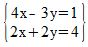# Solving Simultaneous Linear Equations - Quiz & Worksheet

Instructions:

question 1 of 3

### If we have four variables, then how many equations will we have in our system of linear equations?

Create Your Account To Take This Quiz

As a member, you'll also get unlimited access to over 84,000 lessons in math, English, science, history, and more. Plus, get practice tests, quizzes, and personalized coaching to help you succeed.

Try it risk-free for 30 days. Cancel anytime

### 1. Which variable in which equation needs to be eliminated in order to solve this system using Gaussian elimination?### 2. Solve the following system of equations.Create your account to access this entire worksheet
Quizzes, practice exams & worksheets
Certificate of Completion
Create an account to get started

This quiz and attached worksheet will help gauge your understanding of how to solve simultaneous linear equations. Topics you will need to know to pass the quiz include identifying linear equations and solving systems using Gaussian elimination.

## Quiz & Worksheet Goals

Use these assessment tools to:

• Identify number of equations in a given system of linear equations
• Solving given equations
• Eliminating variables in given equations

## Skills practiced

This worksheet and quiz let you practice the following skills:

• Critical thinking - apply relevant concepts to examine information about Gaussian elimination in a different light
• Problem solving - use acquired knowledge on how to solve simultaneous linear equations practice problems
• Interpreting information - verify that you can read information regarding variables and interpret it correctly

To learn more about how to solve simultaneous linear equations, review the lesson Solving Simultaneous Linear Equations which covers the following objectives:

• Define simultaneous linear equations
• Finding solutions to equations
Final Exam